# Using PWM Drives and Slotless Motors to Optimize Performance

Smooth motion, meaning extremely low position and current loop error while at speed, is important to system performance in many precision motion applications, including industrial and surgical robotics, optical scanning and inspection, and semiconductor equipment. Slotless motors offer smooth motion because of their inherent zero cogging design. These motors, however, have extremely low inductance because of their lack of iron in the magnetic field. Low inductance can generate undesirable side-effects such as coil heating from PWM current ripple.

This article looks at how to configure a low inductance motor system to decrease current ripple and reduce position error while under motion. Configurations include PWM rate adjustment, incorporating inductors to each motor phase, and tuning the current loops. Certain background regarding motors and driving inductive loads with a PWM drive will be described to set the stage for a detailed discussion of how to improve a slotless motor system.

## Motor Concepts

### Slotless vs. Slotted

"Slotless" rotary motors do not possess protruding poles (or salient iron teeth), making them physically look and perform differently than a regular "slotted" motor. Rather, slotless motor phase coils are typically form wound and inserted in the stack as opposed to being wound around the teeth of a slotted motor. In contrast to a slotted motor where the rotor's magnetic poles are attracted to the steel "teeth", the absence of iron teeth in a slotless motor makes it have no naturally occurring torque cogging positions.

The stable locations where a rotor of a brushless dc (BLDC) motor aligns with the stator teeth is known as a "cogging" position. The servo system must push its way through these positions so as to rotate the motor. For this reason, slotless motors are favored when smooth motion is critical. There are no cogging forces that the servo must push through, which results in less position error while at speed. For linear motors, the ironless linear motor is comparable to the rotary slotless and the linear iron-core motor is similar to the slotted rotary.

### Motor Poles

The number of magnet poles (and pole pairs) is a key characteristic of a brushless dc motor. The number of pole pairs (N/2), where N is the number of poles, defines the number of electrical cycles in one mechanical revolution. This is sometimes known as the "electromagnetic gear ratio". When in motion, the electrical spatial frequency can be thought of in time as electrical cycles per second or Hertz (Hz). This frequency is the main parameter for which the current loop must be adjusted appropriately.

### Inductance

Any energized inductor has a voltage drop related to the time-varying current through the inductor, (V = L * di/dt, where V is the voltage drop across the inductor, L is in the Henrys and di/dt is the time rate of change of current flowing in the circuit). Alternatively, one could state the time rate of change of current as a function of the voltage across the inductor and its inductance, (di/dt=V/L). This formula is used later to calculate the current ripple formed from the PWM drive. The inductance of slotless motors tends to be a lot less than a slotted motor because the inductance is proportional to the permeance (ability to allow magnetic flux lines to pass) of the coil's magnetic circuit. The presence of iron in the magnetic circuit, acting as a very permeable path for the magnetic flux, makes the circuit permeance much higher. Thus, slotted motors with iron teeth to conduct the magnetic flux have a higher inductance than slotless motors.

## PWM Drive Voltage and Inductive Loads

### RL Load and Voltage PWM

Pulse Width Modulation (PWM) of the bus voltage to regulate the average voltage applied across the motor phases is a typical way to drive motors. PWM motor drives are very efficient and very cost effective versus linear drives. A PWM drive creates the preferred DC current by varying the quantity of on and off time (duty cycle) of the applied voltage. When PWM is used to run a motor, which has resistance and inductance, a dynamic current waveform is created based on the time constant of the RL circuit.

Figure 1 illustrates how current will rise and fall as the PWM voltage is applied across an RL circuit. Here, the PWM is at 50% duty cycle, and the RL time constant is less than the half period of the PWM rate, so the current is reaching its maximum, and minimum, before the next voltage transition. The average current illustrated is about 4 amps with 7.5 amp peak to peak of ripple.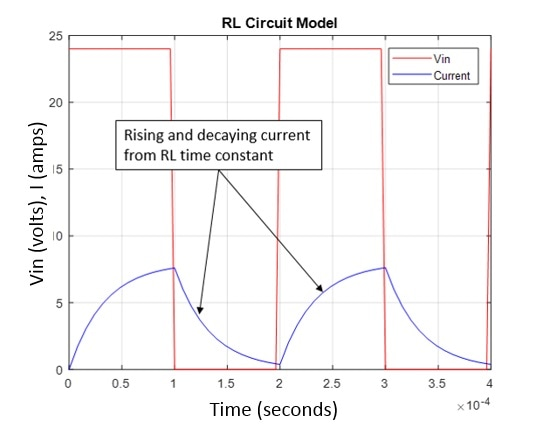Figure 1. Applied PWM voltage and subsequent current in a motor.

### Duty Cycle and Average Current

To create more or less average current, the PWM duty cycle is changed. For example, the maximum current would be available if the duty cycle were 99.9% and conversely, the minimal current available when the duty cycle is at 0.1%. Figures 2, 3, and 4 signify 50, 1, and 99% duty cycles, respectively.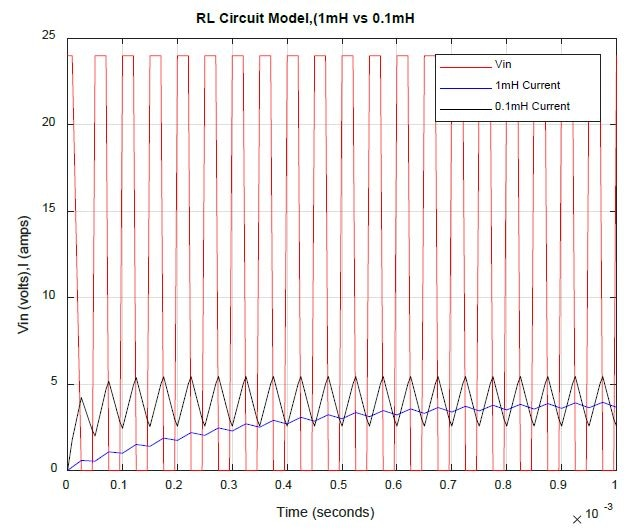Figure 2. 50% PWM duty cycle.

The 50% duty cycle is the worst case for current ripple. The current flowing in the coil has an equal amount of time to build up and decay, making the peak to peak ripple the worst case. For low inductance motors, the peak to peak ripple can be a significant percentage of the average value. Figure 2 for a 50% duty cycle, illustrates a 3 ohm, 0.1 mH motor, having 2.85 amps pk-pk and a 4 amp average. The blue curve, signifying a motor with a more typical inductance of 1 mH, is displaying a slow build up to a steady state current.

This reaction is caused by the larger RL time constant of resistive and inductive load. The 1% duty cycle is illustrated below. In this case, current ripple for low inductance motors is considerably lower than the 50% duty cycle case, yet it is still a large percentage of the average. In the 1% PWM case illustrated in Figure 3, the 3 ohm, 0.1 mH motor has 0.12 amps pk-pk and an average of 0.1 amps.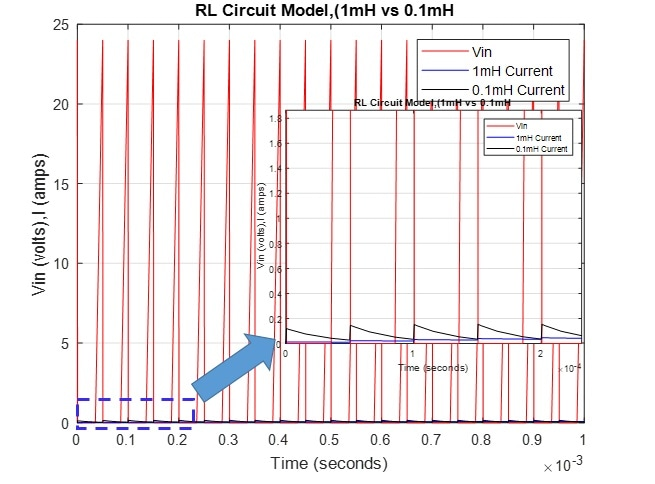Figure 3. 1% PWM duty cycle.

The final case (presented in Figure 4) is the 99% duty cycle. Here, the peak to peak current ripple is the same as the 1% case, but versus its average, it is a much smaller percentage.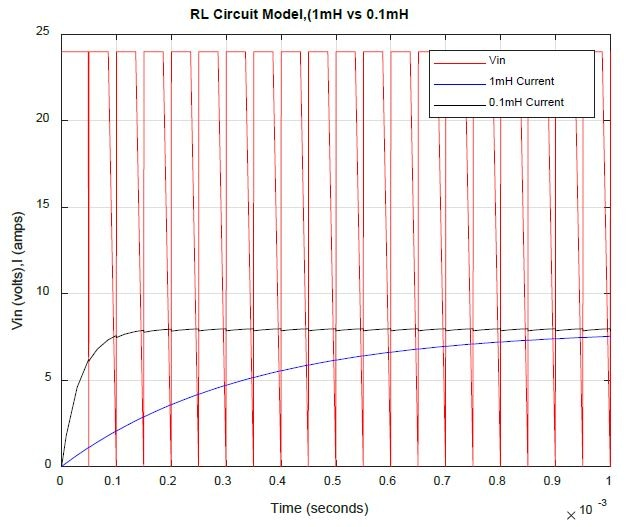Figure 4. 99% PWM Duty Cycle.

### PWM Rate and Current Ripple

Motor power dissipation (motor heating) is frequently a concern for precision mechanisms. Based on the application, current ripple formed from PWM drives and low inductance motors can be a key contributor to motor heating. In the 50% duty cycle case illustrated in Figure 2 above, the peak to peak ripple is nearly as large as the average current. For a motor with 1 amp of average current, and 0.6 amps of peak to peak (proportionally similar to the case illustrated in Figure 2), the RMS of the total current is 1 + 1/sqrt(3)*0.3 = 1.17 amps.

This is a 17% increase over the average current. In motor power dissipation, the current is squared, so 1.17 amps squared becomes 1.37 amps and power dissipation increases by 37%. Thermal resistance is the measure of temperature rise per watt, so a 37% increase in dissipated power will equate to a 37% increase in temperature of the motor coil.

A simple way to decrease the dissipated power is to boost the PWM rate. Not all drive manufacturers will recommend doing this, as it puts more thermal load on the drive devices, which has probably not been assessed at the proposed PWM rate and motor load. It is best to discuss these issues with the drive company's Applications Engineers and inform them of any motor power dissipation apprehensions when driving a low inductance motor.

The formula for voltage drop across an inductor (described previously) can be used to calculate the maximum quantity of current ripple in a motor. In this calculation, care must be taken in using the correct voltage. For instance, when assessing current ripple at very low speeds, one can typically ignore Vbemf.

When the back emf becomes greater than 10% of the bus voltage, it has to be subtracted out to get an accurate current ripple calculation.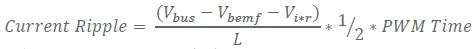Equation 1. Current Ripple from PWM Rate and Vbus

In this section, current ripple versus PWM rate is compared using a low inductance motor (0.1 mH). Figure 5 illustrates how doubling the PWM rate will half the peak to peak current ripple. The reduction in ripple is due to the reduced time available for current to rise and fall based on the RL time constant.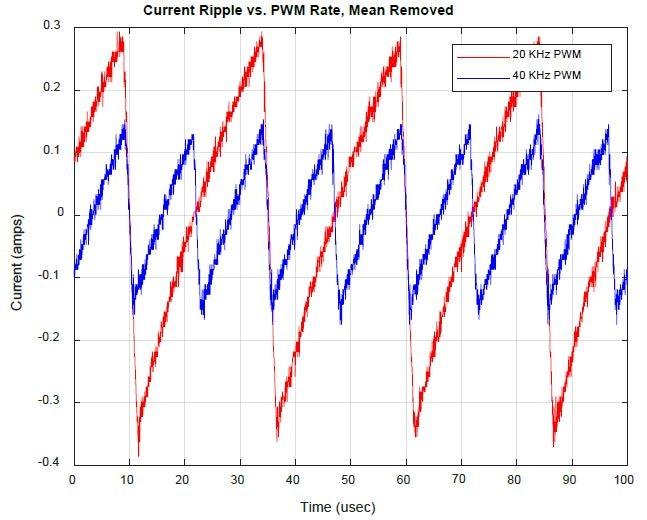Figure 5. Current Ripple vs. PWM for 1 amp command (Red- 20 KHz, Blue- 40 KHz).

15-20 KHz is a common PWM rate found on most drives. 30-40 KHz is available from a number of manufacturers but requires significant arrangement and discussion to implement and is frequently not clearly documented. 60-100 KHz is available from a small number of suppliers and can greatly decrease current ripple. Figure 6 is a compilation of locked rotor test data from three common drive manufacturers. A locked rotor test means that the rotor is not permitted to spin while a constant current is being commanded to the drive.

A current probe was utilized across one of the motor phase leads. The rotor was rotated manually to locate an electrical angle such that the phase current for the probe was at its maximum. The data evidently displays three key points. Observation 1 is that higher PWM rates result in lower quantities of current ripple. Observation 2 illustrates how all three drive companies have similar performance. The third point, which is less apparent, is that one of the three drive companies did not offer an 80 KHz PWM rate. PWM rates are the parameter that should be talked about with the targeted drive manufacturer when using slotless motors.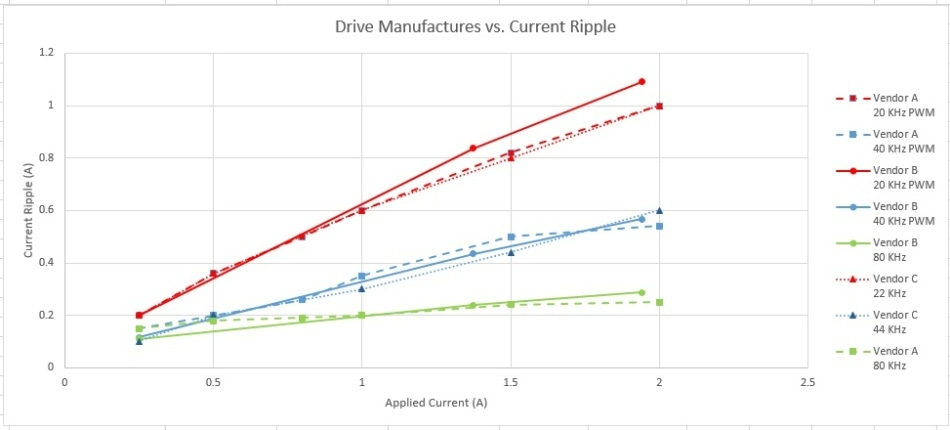Figure 6. Current Ripple vs. Drive Company vs. Drive Level.

If a drive does not support medium to high PWM rates, meaning greater than the standard 15-20 KHz range, and current ripple (motor heating) is still an issue, integrating inductors in series with each motor phase is an option. The integrated inductance does come with integrated resistance and a loss of efficiency, but it can greatly decrease current ripple and make the motor run cooler. Figure 7 illustrates how doubling the inductance accomplishes the same ripple and doubling the PWM rate. When using add on inductors, care must be taken in properly sizing the inductors by current capability or power dissipation.

Usually, this is only a feasible option with low current drives as inductor sizes can swiftly exceed motor sizes as the vital current levels increase. Other factors related to add on inductors is that they require new current loop tuning, as the RL pole in the plant will have moved, and require extra cabling for connecting to each motor phase. In a number of applications, this option is just too complicated because of space limitations.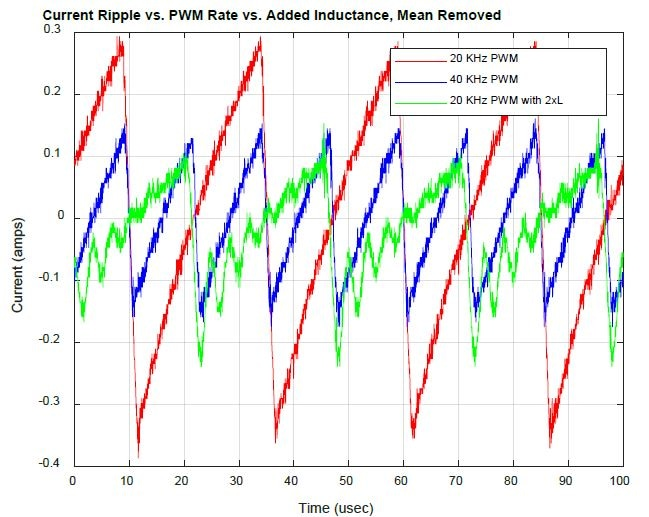Figure 7. Current Ripple with Added Inductance.

## Control Architecture

### Inner and Outer Loop Architecture

Rotary motion control is usually realized through the use of at least two nest control loops – an outer position loop and an inner current loop, (see Figure 8). A velocity loop is sometimes incorporated between the fast current loop and slow position loop.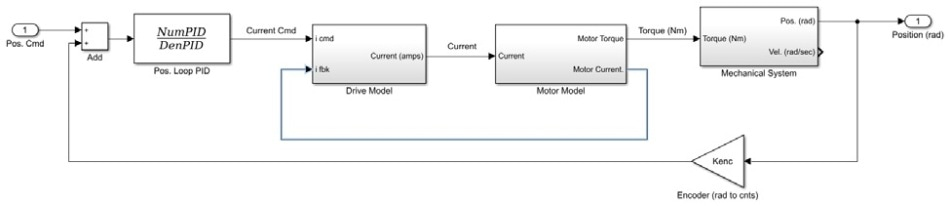Figure 8. Nested motion system architecture.

In the nested loop architecture, the current loop's function is to supply current based on the torque required to make the outer position loop error a minimum. Normally the current loop bandwidth is 10 to 20 times higher than the position loop bandwidth, putting it near or greater than 1 KHz. Tuning of these two servo loops has a direct effect on how well slotless motors act in any motion system.

### Target Current Loop Bandwidth

Before any current tuning starts, the highest operating speed and pole count must be used to calculate the electrical cycle frequency for the system. The formula is just; N/2 * RPM/60, where N is the number of poles and the units are electrical cycles per second. The current loop gains must be tuned so that the bandwidth is at least 2 to 3 times more than the electrical cycle frequency, allowing the drive to create phase current instantly, as the commutation algorithm switches the applied current into the correct phases throughout a mechanical revolution.

### Current Loop Tuning

Two phases of a BLDC motor can be signified as a simple RL circuit, which when represented in the LaPlace domain takes the form of a 1st order system. It turns out, however, that this RL, 1st order transfer function alters when the effect of the motor's back emf is considered. If the effect of back emf is simulated, the "Plant" for the current loop becomes the whole block diagram illustrated in Figure 9 below. As more back emf is allowed to flow, the transfer function changes from a first order shape, to a second-order transfer function that transitions from +1 slope to -1 slope in magnitude and +90 to -90° in phase (see Figure 10.).

This intense change in the transfer function would generally be considered harmful to a fixed PI control law. Here, however, the change is towards stability, not away from it (meaning phase is integrated, not removed), making the loop stable when the motor is at rest, and while moving.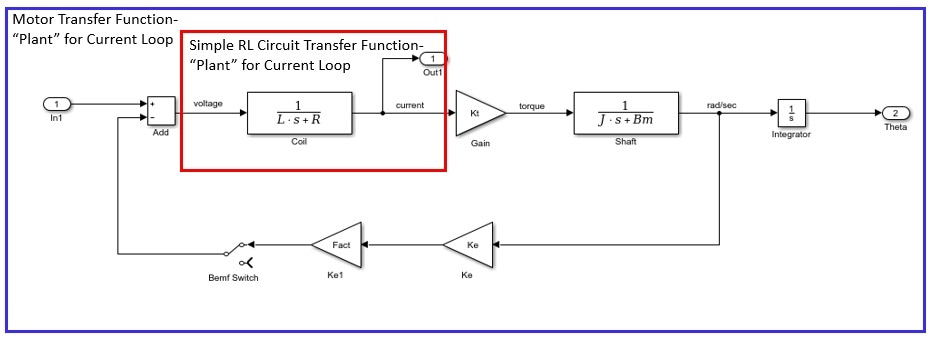Figure 9. Block Diagram of the motor transfer function.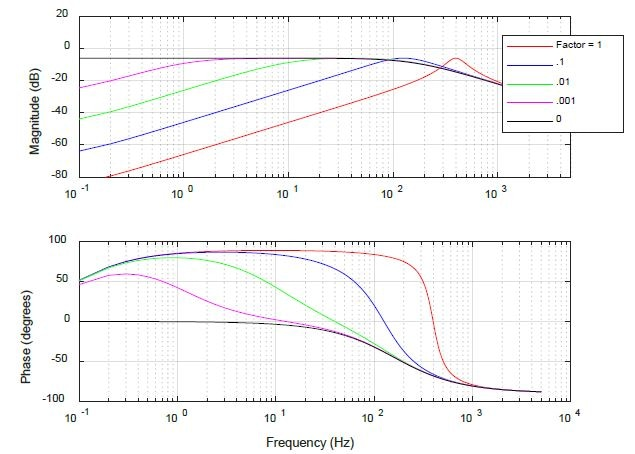Figure 10. Motor Plant Transfer Function vs. Back Emf Factor.

Regulating the plant transfer function illustrated in Figure 10 requires only a Proportional + Integral (PI) controller with an extra first order low pass filter on the current sensor. The low pass filter is usually at a fixed value in the drive software, thus the user doesn't usually tune this value. Tuning the current loop is nearly always done with a step response tool within the drive software. In certain cases, a higher fidelity tuning technique is required to get the absolute maximum performance out of the current loop. In that case, tuning is recommended to be done in the frequency domain using Bode Plots. First current loop tuning within the time domain will be discussed, then move into some more advanced tuning in the frequency domain using Bode Plots.

Figure 11 shows simulated data from a low inductance slotless motor. The plot has many curves, each with different PI gains. As the PI gains are increased, the response gets closer and closer to the commanded step in current. The green trace would be considered by many to be the best combination of response time and overshoot. In certain applications, however, more performance is required, and overshoot is a metric that can be relaxed at the benefit of improved disturbance rejection. Having a small quantity of overshoot, without ensuing oscillation is a stable gain set and is the maximum gain possible for the system.

Many drive manufacturers do not propose this level of tuning, as in certain cases it can interact with high-frequency structural resonances and produce an audible tone, or worst case, instability. Based on the application, however, this level of tuning can give the system the additional performance it requires. In the Section “Advanced Current Loop Tuning”, optimal I gain tuning is discussed and it is revealed how higher I gain values yield improved disturbance rejection.

### Position Loop Tuning

Position loop tuning is critical in establishing how a precision mechanism or motor system moves and settles. Tuning the position loop, however, is not directly impacted by the motors' inductance. The "Plant" for the position loop is really the inner current loop plus the inertia of the motor shaft (or moving structure) and any structural dynamics it may have. The challenges of position loop tuning are connected to these structural dynamics and any time lags between the sensor and the motor. These concepts are not covered here, as this article is focusing on tuning for low inductance motors.

In the Section “Control Architecture”, time domain tuning of the current loop's PI gains was discussed. In that section, it was illustrated how P and I gain impact the time response from a step input of current. This section shows how the P and I gains shape the loop transfer function in the frequency domain.

### Frequency Domain Tuning

Position and integral control are signified in the LaPlace domain as, where A = Ki/Kp and Gain = Kp. This form of PI control is crucial to note as it tells the control designer that at frequency "A" the integrator turns on (or off). Turning the integrator "on" at a higher frequency means that the gain will be higher for all frequencies below A (see Figure 13). The beauty of frequency domain tuning is that the control designer can visually see how the Ki gain just increases loop gain below the corner frequency A, and not above. When tuning in the time domain, this impact is not observable. Increasing just low-frequency gain is important when the system has high-frequency resonances above frequency A that might have Gain Margin sensitivities.

In control design, one must frequently make decisions and tradeoffs based on the applications needs. Here, the benefit one gets from increasing low-frequency gain causes lost phase near the targeted servo bandwidth. Turning the integrator on at a higher frequency means the quantity of phase margin at the bandwidth is decreased. Figure 13 illustrates how the phase from PI control alters with frequency and specifically, one can see that at the targeted bandwidth (the green circle), there is about 15° of phase loss from the 10xKi case versus the Ki gain case.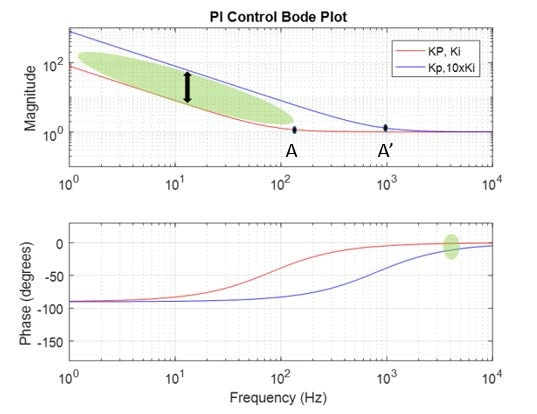Figure 11. I gain effect on Bode Plot.

To finish this discussion of frequency domain tuning, a discussion on how Kp gain affects the loop transfer function is needed. Above it was stated that a PI controller is signified as, where A=Ki/kp and Gain = Kp. In Figure 14, one will notice that if Kp is changed, both the integrator frequency A and the high-frequency gain value are also changed. The combined effect of a change in "Gain" and the corner frequency A, basically keeps low-frequency gain unaffected and boosts high-frequency gain. It also impacts the phase curve in that more phase margin would be available since the corner frequency has been decreased.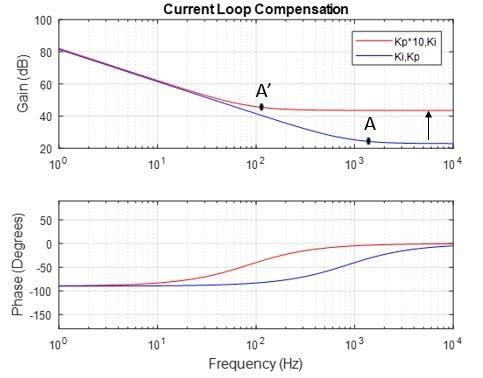Figure 12. Changing only Kp.

### Example Using High I Gain

This section shows an example of how increased I gain compensation can be used to enhance current loop performance. As stated earlier, an ironless linear motor is in many ways comparable to a rotary slotless motor. Below is a streamlined image of the workings of a linear motor.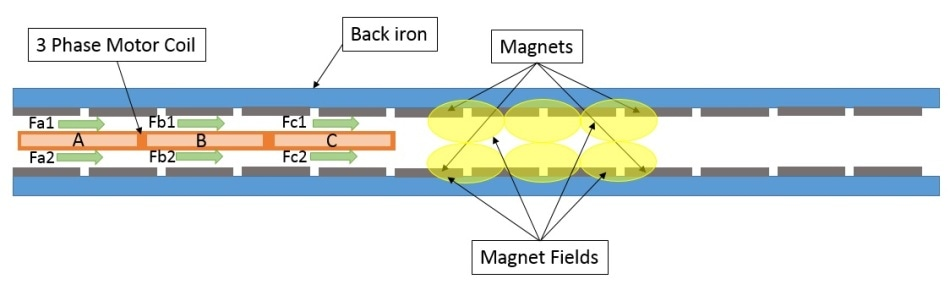Figure 13. Linear Ironless Motor.

In this image, forces are produced from the interaction of the current running through motor phases A, B, and C and the corresponding magnetic field because of the individual magnets. This interaction of current and magnetic field is captured in the motor parameter Kf (N/amp). Each separate magnet, however, has marginally different magnetic strength causing the magnetic fields to correspondingly differ in strength. This, in turn, causes the motor's Kf to differ in strength as a function of position and eventually causes the forces Fa1 through Fc2 to differ in strength with position. Other contributors to position-dependent force variability are magnet spacing, motor coil to coil spacing, and motor coil turn spacing. All of these integrate to create a varying Kf with position.

As mentioned previously, the motor parameter that captures this conversion of applied current to force is the force constant of the motor (Kf). When using SI units, the back emf constant is numerically the same as the force constant. So, as the coil moves through the varying magnetic field, the back emf constant also varies, thus generating a fluctuating back emf voltage. The varying loss of voltage as a function of position is subtracted from the bus voltage, and the remainder is applied across the coil to create current. The block diagram below illustrates where the back emf loss is accounted for in relation to the current loop and a simplified RL motor model. The current loop's job is to keep the preferred current correct while any voltage disturbances are occurring. Here, as the coil moves through the varying magnetic fields, there is a voltage disturbance (in the form of back emf) that the current loop must correct for. In the block diagram, this is captured through at the sum block just before the coil model.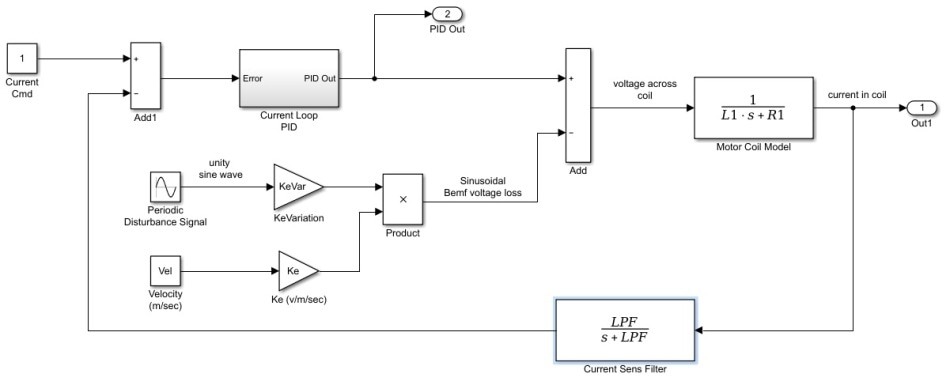Figure 14. Block diagram for current loop and motor with varying Kf (ke).

The frequency of the voltage disturbance is important. For slow speeds, the frequency is also slow, as the frequency is the linear speed in mm/second divided by the magnetic pitch (mm/pole pair or electrical cycle). The units here become electrical cycles per second or "Hz". At the low speeds (and low frequencies), the loop gain is very high (see Figure 17). In these regions, it may be hard to see any advantage of optimal integrator tuning because the gain is so high that improvement may be in the noise of the measurement. At higher speeds (and frequencies), however, maximizing I gain could result in a two or three times improvement in current ripple from varying Kf.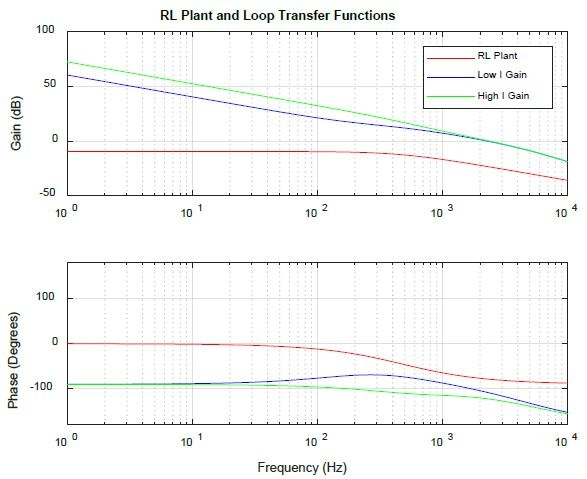Figure 15. RL Plant and Loop Transfer Function plot for low and high gain current loop.

For this example, a 200 Hz sinusoidal signal was injected into the voltage summation block. The amplitude of the sinewave was fixed using a Ke value of 10 volts per m/second, at a speed of 0.5 m/second and a variation of 10% of that value. What this means is that Kf (or Ke), will differ +/- 10% at a frequency of 200 Hz. This is used to signify how a linear coil moves through a motor track with differing magnets strength. The varying Ke will cause a fluctuation at 200 Hz of the back emf component of coil voltage. This fluctuation will act as a disturbance to the voltage being applied to the coil.

Figure 17 illustrates the two Loop Transfer functions that were assessed. Both have a 0 dB crossover frequency of approximately 2 KHz (bandwidth) but have different low-frequency gains because of the different I gain used. At 200 Hz, the gain difference is around 10 dB, which is a factor of 3. The net benefit of the 3x in gain is illustrated in Figure 18 where the higher Ki case is clearly performing better by about 2 to 1. It should be noted that if the back emf voltage model were applied for this example, the red curve would be shaped like the red trace of Figure 10, but with a peak at 400 Hz, and the slope of the green and blue curves would flat below 400 Hz, separated in amplitude by the same amount as illustrated here.

Drive companies will normally not suggest this level of advanced tuning as it tends to decrease phase margin of the current loop. The amount of acceptable Phase Margin is not the same for every application. In mechanical systems, closing loops with 30° in margin is common practice, while in electrical engineering disciplines, like op-amp design, loops are closed with a minimum of 60° margin. The I gain optimization detailed here can become a very beneficial tool for the right application. The higher level takes away from this discussion, however, is that when tuning a control law to reject tonal frequencies, gain is what is vital, not bandwidth. As presented above, two systems with the same bandwidth perform a lot differently against a tonal disturbance.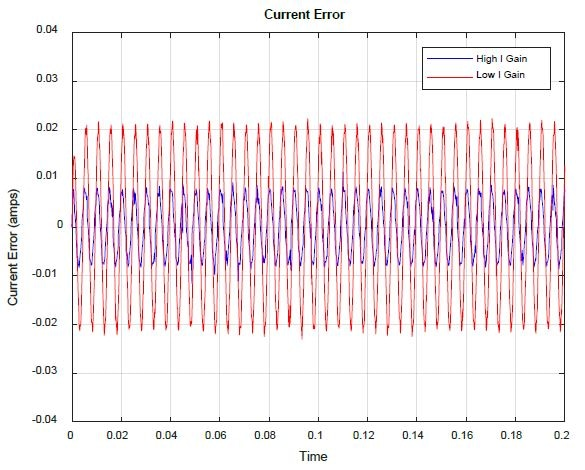Figure 16. Current Loop Error vs. I gain.

## Summary and Conclusions

Enhancing the performance of a slotless motor driven by a PWM voltage drive requires knowledge of how a resistive and inductive load acts under varying applied voltage. Understanding the tuning principals of the proportional and integral controlled current loops is also vital for making the most out of the slotless motor system.

This article covers some elementary motor and drive concepts including inductance, pole count, and PWM voltage. The difference between a slotless and slotted rotary motor was discussed and it was noted how slotless motors usually have low inductance. The fast RL time constant of a slotless motor, along with a PWM drive, creates current ripple that can be a great contributor to dissipated power in a motor. Different methods to decrease current ripple, including PWM rate, and added inductance were also discussed. Increasing the PWM rate or incorporating inductance were both revealed to be extremely effective in reducing current ripple. Three common PWM drive manufacturers were analyzed and compared for current ripple and all were found to be similar in their performance, but not all offered an 80 KHz PWM rate.

Time domain current loop tuning was looked at and it was revealed how current loop proportional and integral gains impact rise time and overshoot. Advanced frequency domain tuning was discussed where it was shown how P and I gain shape the gain and phase vs. frequency curves. The take away from frequency domain tuning is that changing I gain modifies only the low-frequency part of the gain curve, so as to not unfavorably affect any resonances in the system at a higher frequency. This advantage of increased low-frequency gain is at the expense of lost phase at the targeted bandwidth, so care must be taken to not destabilize the loop when enhancing I gain.

The theory of maximizing I gain was taken to a simple motor example, where a voltage disturbance was an input to the commanded voltage across a resistive and inductive load (i.e. a motor). It was demonstrated that the current error was decreased by a factor of 2 with optimizing I gain tuning. Additive inductance, PWM rate, and current loop tuning are all "tools" one can use to improve the performance of slotless motors with PWM drives. Using these methods will improve the inherent smooth motion benefit of a slotless motor.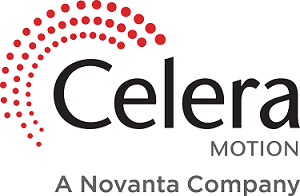This information has been sourced, reviewed and adapted from materials provided by Celera Motion.

## Citations

• APA

Celera Motion. (2020, October 23). Using PWM Drives and Slotless Motors to Optimize Performance. AZoSensors. Retrieved on October 25, 2020 from https://www.azosensors.com/article.aspx?ArticleID=1019.

• MLA

Celera Motion. "Using PWM Drives and Slotless Motors to Optimize Performance". AZoSensors. 25 October 2020. <https://www.azosensors.com/article.aspx?ArticleID=1019>.

• Chicago

Celera Motion. "Using PWM Drives and Slotless Motors to Optimize Performance". AZoSensors. https://www.azosensors.com/article.aspx?ArticleID=1019. (accessed October 25, 2020).

• Harvard

Celera Motion. 2020. Using PWM Drives and Slotless Motors to Optimize Performance. AZoSensors, viewed 25 October 2020, https://www.azosensors.com/article.aspx?ArticleID=1019.